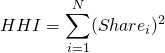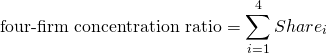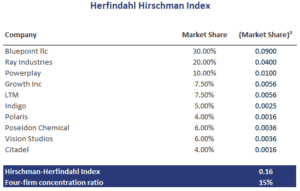# Herfindahl Hirschman index (HHI)

The Herfindahl Hirschman index or HHI index is an important measure of market concentration. It is a competitiveness measure that is used extensively to assess the amount of market concentration in a particular market. The measure was introduced by Albert Hirschman and Orris Herfindahl, although it is sometimes referred to simply as the Herfindahl index or H index.

Another example of measure that capture market concentration is the N-firm concentration ratio. This ratio, usually calculated for the top 4 firms, has a number of drawbacks. This explains why the HHI is more commonly used than the four firm concentration ratio. Nevertheless, we also provide the four firm concentration ratio, discuss how to calculate CR4, and to contrast it with the HHI index

On this page, we discuss the Herfindahl Hirschman index formula, illustrate how to calculate and interpret the herfindahl index, and finally provide a Herfindahl index calculator as well as a four firm concentration ratio calculator in Excel at the bottom of this page.

## Herfindahl Hirschman definition

The HHI index is calculated as the sum of the squares of the market shares of the largest firms in the market. The measure ranges from 0 to 1. Sometimes, however, whole percentages are used in the calculation, in which case the index ranges from 0 to 10,000 points.

Next, let’s turn to HHI calculation by applying the formula to an Herfindahl Hirschman index example.

## Herfindahl Hirschman formula

The formula for the HHI index is the following## Four firm concentration ratio formula

A related measure that also measures market concentration is the four-firm concentration measure## Herfindahl Hirschman limitations

There are a number of important limitations to the HHI index. First, the HHI index does not consider barriers to entry. It could be that a market is highly concentrated, but entry barriers are low. In that case, market power may be very limited. Second, we have to choose the ‘largest companies’. This means that different researchers may come up with different HHI values for the same market.

## Herfindahl Hirschman calculator

Next, we illustrate the use of the HHI as well as the four-firm concentration measure (CR). Let’s assume the following set of firms, with the sum of the market shares summing to a 100%. Then, the HHI and CR4 are the following### HHI calculation

How to calculate Herfindahl index in Excel? We illustrate the calculation and include a simple four-firm concentration calculator in the following spreadsheet: Herfindahl Hirschman Index template